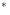Sie sind hier: ICP » R. Hilfer » Publikationen

# Index

• absolute time
• algebra
• Aristotelian instant 2, 3
• Aristotle
• beginning
• Bochner-Levy diffusion
• C-algebra
• character 4, 5, 5, 7
• recurrent
• classification parameter
• Cole-Davidson
• composite time evolution
• conservative system 12, 5, 5
• continuous time random walk 12, 12
• convolution 1, 8, 8
• semigroup
• CTRW
• Debye
• dielectric function
• dielectric relaxation
• diffusion
• Bochner-Levy
• fractional
• Montroll-Weiss
• discretization 6, 7, 8
• dissipative system
• dynamical rule
• dynamical system
• fractional
• dynamics 1, 1
• fractional
• excess wing
• flow
• Fokker-Planck
• fractional 1, 1, 10, 8, 8
• derivative
• integral representation
• differential equation
• diffusion
• dissipative system
• dynamical system
• dynamics
• master equation
• time evolution
• generator 10, 11
• infinitesimal 10, 11
• glass 11, 11
• glass transition
• glassy relaxation
• glycerol
• group 3, 9
• one-parameter
• Havriliak-Negami
• infinitesimal generator 10, 11
• invariant measure
• ergodic
• irreversibility
• irreversibility problem
• normal
• reversed
• Kac’s theorem
• KWW
• master equation
• fractional
• measure 5, 5, 6, 8
• invariant 5, 5, 6
• preserving system
• preserving transformation 5, 6, 7, 8
• induced
• Kakutani’s
• Mittag-Leffler
• Mittag-Leffler function
• binomial
• mixed state
• Montroll-Weiss diffusion
• Newton
• observable 3, 3, 4
• time evolution of
• phase space 5, 6
• Poincare’s recurrence theorem
• probability measure 5, 7
• induced
• space
• propylene carbonate
• random walk 12, 12, 12
• recurrence 6, 7, 8
• recurrence time 6, 7
• recurrent character
• relaxation
• Cole-Davidson
• Debye
• dielectric
• Havriliak-Negami
• Kohlrausch-Williams-Watts
• KWW
• rescaling constants
• semigroup 3, 9
• state
• subordination
• susceptibility 11, 11
• dielectric 11, 11
• frequency dependent 11, 11
• system
• conservative 12, 5, 5
• dissipative
• dynamical
• theorem
• fractional dynamics
• Kac’s
• local limit
• Poincare’s recurrence
• Riesz representation
• time
• absolute
• arrow
• flow
• recurrence 6, 7
• waiting
• time evolution 3, 3, 4, 4, 5, 6, 8